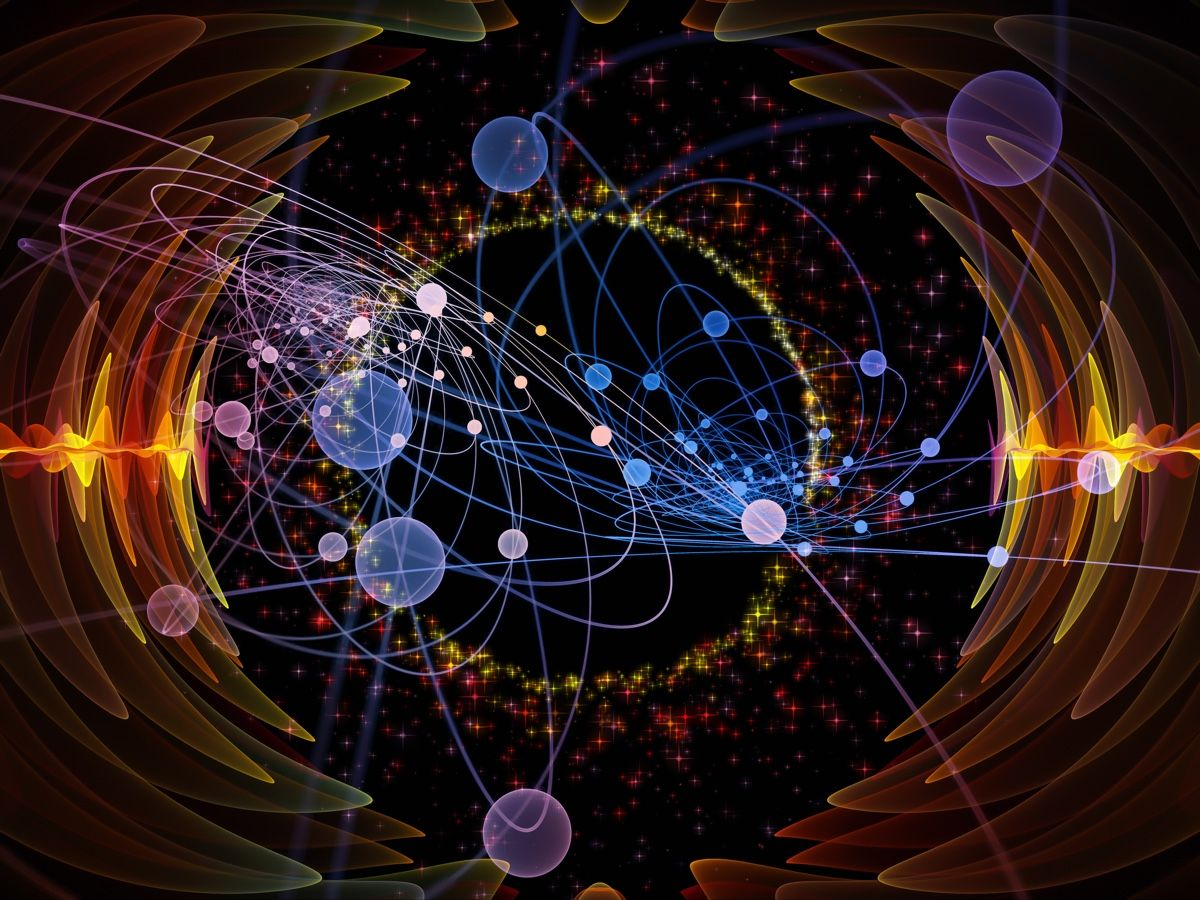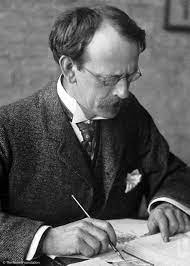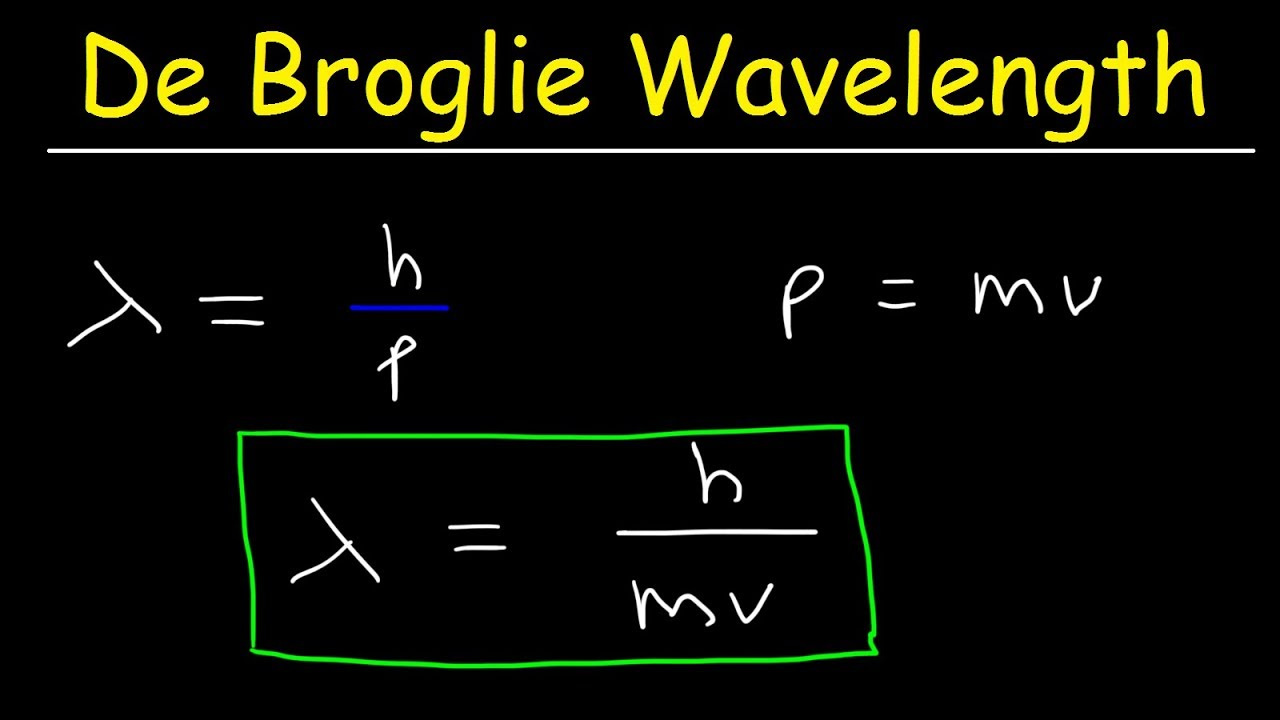# The Wonder of Quantum world

## To understand these manipulations of the quantum world, we need to understand the basics of quantum mechanics.In the world of tiny particles, our conventional thinking stumbles. This is what we all heard. But why? And where is the problem or so much objection? But many people say, don't go to understand the matter of a very small or quantum world, you won't be able to. Premature loss of head. What is the need to run behind what is not understood or what?

Is it a matter of work at all? To understand these manipulations of the quantum world, we need to understand the basics of quantum mechanics. Mechanics is the spread of many theories, where the mathematical description of the motion of an object is found at the coincidence of all the working balls.When and where an object will go under a ball is known by the calculation of its hadith mechanics. Mechanics is a big deal. There are many theories in it, many people's small streams of thought together create a big river. Then the river speeds up, people get immense pleasure by bathing in that river of thoughts. This joy is the joy of knowing, this joy is the joy of understanding nature exactly.

Quantum mechanics is one of the greatest monuments of the twentieth century. Such an unprecedented combination of thought and mathematics rarely happens. What is quantum mechanics? This is how the science of microscopic electrons-protons will be arranged in atoms, how a tiny particle will behave under an imposed ball, what will be its trajectory all these are discussed here.

The purpose of quantum mechanics is to explain the forces acting in the world of tiny particles and the behavior of tiny particles under them. As can be seen, this mechanics speaks of events that are unconscious. If a tennis ball is thrown against the wall, it is easy to guess where it will hit. But it is absolutely certain that the walls will not be blown out. Again its speed and mass can be measured simultaneously.

But quantum mechanics says that if this tennis ball were the size of an electron, none of it would be. Then you will see all the strange things. In a tiny quantum world, tennis balls, walls everything will be tiny. So in this world, the smallest tennis ball will create a deflection pattern on the so-called quantum wall, it can break through the wall. Even its position and momentum cannot be measured simultaneously. In addition this electron shaped tennis ball will also vanish.

In its place will be a bunch of waves, the squares of which tell the probability of getting the ball at some point. These events are contrary to common sense. So it is better to keep common sense while learning the rules of quantum world. It is better if only mathematical reasoning can be followed blindly. The decision that will be made in the end, of course, matches the observation. If not, people would not believe in quantum mechanics.Quantum mechanics began in 1900, with the proposal of Max Planck. Soon after Planck's theoretical proposal, Niels Bohr developed a model of the atom to explain the Balmer series of spectra emitted by the hydrogen atom. In that model the orbit of the electron is broken. That is, electrons can no longer exist outside of a few approved orbits.

Thus the orbit of the electron is quantified. This is called the Bohr model. The Bohr model was explaining everything fairly nicely. However, there were many questions, and everything did not match. Finally, in 1925 and 1926, the journey of quantum mechanics began with the special contribution of two scientists.

Werner Heisenberg was a young German and a gifted student. He was concerned about the spectrum of atoms. At that time spectroscopy was very important. This is because the frequency of the spectral line could be measured very accurately and the science of spectroscopy was trying to reveal many details about the nature of these observations. Physicists were also engaged in the origin and interpretation of different lines of the spectrum.

So chronology was highly valued in the world of modern physics. For example, the Bohr model has to be used to explain the origin of the Balmer line in the spectrum of hydrogen atoms. Heisenberg was deeply concerned about this.

He wanted to present a general explanation of the light religions so that the inner structure of the object could be better known. To recover from an awkward fever, he went to an island in the northern ocean. At that time he sat down with these complex calculations. As it turned out, he came up with all the mathematical terms known as matrix. A matrix is ​​a kind of table of numbers.

The multiplication of the matrix is ​​different from the conventional multiplication. They are non-negotiable. That is, as we see in general 2 × 3 = 3 × 2, the matrix but not so much. The product of two matrices is ab-ba ≠ 0. That is, in terms of multiplication of the matrix, which term comes first is important. Because of this unchanging religion of mathematics, everything we see in quantum mechanics is irrational.

As a result of such multiplication, there are some mutually complementary physical quantities which cannot be measured together. It is not possible to measure the position and momentum of such particles with equal certainty. This is the essence of Heisenberg's policy of uncertainty.

This is why many people refer to this mathematical dealing with the matrix as 'matrix mechanics'. Today's science students learn addition-subtraction-multiplication of matrices in high school or higher matriculation in graduation class. But that was not the case in the 1920s. In the old days wave analysis was more popular. Because a few days ago Maxwell published his famous electromagnetic theory. Show in that theory, radiation moves forward in waves.

On the other hand, Mr. Heinrich Hertz also discovered electromagnetic waves. So wave science was a big favorite subject then. Partial differential equations are associated with waves. A complementary analysis with the help of this waveform came after Heisenberg. Erwin Schrödinger, the inventor of the second mathematical formula.

Erwin Schrödinger was born in an aristocratic house in Austria. He followed in the footsteps of Louis de Broglie. The idea of ​​The Broglie was that if a wave of flickering light showed particles, then any object must have waves. Just as Einstein's law of energy (E = hv) is quantized, so is the wavelength formula of The Broglie.

The main characteristic of quantization is the presence of ‘h’ in the equation. The value of h-quantity known as Planck's constant is very small (6.626176 × 10 ^ -34 joules / second). Quantum mechanics works in the world of tiny because its value is very small. If its value was large, quantum uncertainty would affect our daily lives. To know what that would be like, the reader should read George Gamow's book Mr. Tompkins in Paperback.

The Broglie published this theory in his PhD dissertation written in 1924. Researchers at the University of Paris did not understand the strange theory of matter, seeing waves and particles at the same time. Then they swore to Einstein. The degree was awarded to The Broglie shortly after Einstein agreed. Within a few years, evidence was found that the electron beam deflected the solid material with lattice and created an irregular pattern. The experiments were performed by Davison and Germer of the United States and George Thomson of Britain.This Thomson is the worthy son of the well-known JJ Thomson. The eldest Thomson showed that the electron is a particle, and his son showed that the electron is also a wave. Thus evidence of Broglie's theory was found. He was awarded the Nobel Prize in 1929. Broglie's idea was to establish a relationship between the momentum and wavelength of a particle moving freely in space. But to apply it in a more comprehensive case it is necessary to review the interaction of these particles with the applied force or energy. Zeroing the imposed voltage will re-establish the relationship of The Broglie.

That is, it is possible to find a kinetic equation or set of equations that would explain the behavior of wave particles in general in a very wide range of cases, and whose special solution would be The Broglie's equation. This is exactly what Schrödinger did in 1926. He wanted to write a freely circulating wave in the form of the well-known Helmholtz wave-equation. To do that, he used The Bigley's formula and commentary on the principle of uncertainty.

This results in the import of energy and Hamiltonian operators in place of the space-derivative ((d / dx)) and the time variable (d / dt) of the wave-equation. Thus the Schrödinger equation is obtained. The short well-known form is: Hψ = Eψ. This is the basic dynamic equation of quantum theory. It is a partial differential equation and it is very easy for mathematicians to solve.

Unprecedented success was achieved by applying this equation to various real systems. The problem of overcoming the common voltage-barrier is that a particle like an electron can easily break through the walls. It is a tunneling process. Mr. Schrödinger himself was able to solve the Balmer series of the hydrogen spectrum by determining the solution of his equations in the case of hydrogen.As a result, it is possible to show how incomplete Bohr's model was, despite its close proximity to quantum theory. The main problem is that the actual quantification of momentum is not what Bohr thought it was, or in Broglie's law.

‘It is very important that there is no determination of the Schrödinger equation. It's just a guess. We can calculate the various relevant results of these estimates and then compare those results with the test. We see that the success of Schrödinger’s equations is so great that it is not a coincidence. It is, of course, the basic equation that is able to successfully explain the non-relativistic physical phenomena of the microcosm.Shortly after Schrödinger's wave mechanics and Heisenberg's matrix mechanics (Schrödinger published his equations a few months after Heisenberg's publication) Max Born and Paul Dirac proved that these two mechanics were in fact two different mathematical models of the same phenomenon. In fact, they each describe the quantum theory, but their mathematical expression is different.

It becomes a matrix when you write the value of a physical object scattered in waves over space and time in a cluster. In a sense, matrix mechanics is more mathematically powerful, because more mathematical processes can be applied to the matrix. It is also convenient for calculations because the computer code matrix is ​​much easier to understand.

What is the cy-function or wave of Schrödinger's equation? Is it a particle wave? No, Max Burn said it was actually an abstract mathematical construction. Its true meaning appears only when its square is calculated. The square of the cy-function (| ψ | 2) indicates the probability of finding that particle somewhere.

This is known as the Copenhagen explanation. Those who work with quantum mechanics say that it is better to calculate silently (shut up, and calculate) than to know and understand more! Only then will you find a testable solution. Feynman also said that no one understands quantum mechanics.

Understandably or not, quantum mechanics has now become a fundamental and important element of modern education. No one can make a solid-state device without knowing it, no one can understand the speed-nature of that device. So students of electrical engineering also have to learn it.

If you don't know it, you can't understand the bond of the element, so the chemistry student also reads it. There are no Hen branches of Materials Engineering, Photonics, Condensed Matter-Science and Engineering where Quantum Mechanics is not required.